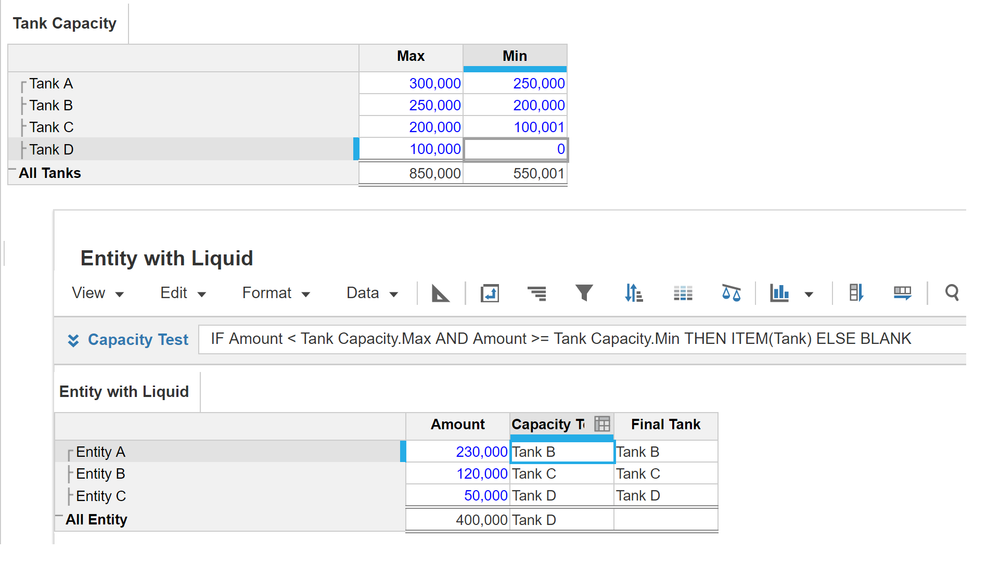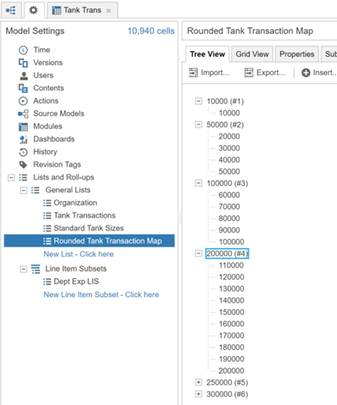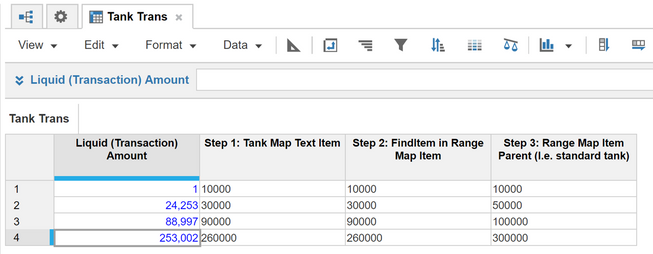# Any Excel equivalent for Match function with Match type 1 or -1?we have Excel Match formulas which looks thru a lookup_array

1) which finds the largest value that is less than or equal to lookup value (match type 1) and

2) which finds the smallest value that is greater than or equal to the lookup value (match type  -1)

Eg. of Usage : Supposing we have tank table for liquid A as below. Need to know TankCateg for smallest  tank which will hold a 150K gallons liquid A. Using match type -1 in Excel Match function, the tankcateg is 3, capacity 200,000.

Liquid Type A Tanks:

TankCateg       Capacity(gals)

1                      300,000

2                      250,000

3                      200,000

4                      100,000

5                        50,000

6                        10,000

We have  tanks sizes and category in a  table as there are many tank categories and liquid type.

How do we do this in Anaplan?

Thank you,

Cheng

Tagged:

•The formula for "Capacity Tank" is shown above.

You'll need to select "Last non blank" for Summary method of "Capacity Tank".

Formula for "Final Tank" is just "Capacity Tank"

•However this is accomplished, it needs to scale.  Specifically, if the number of Liquid A transactions grows (potentially into the millions & up), the method of mapping each transaction to the standard tank size needs to remain relatively constant.  (Combining Transactions with tank sizes in a single module may not scale well if there are a lot of standard tanks & a lot of transactions)

One way to do this (in a way that will scale) is to (dynamically) map Liquid A transactions to a managable map.. that will, in turn, get us to our standard tank.

The first step would be to determine the standard tank mapping increments.  In the example below, I created a simple list that assumes a 10,000 gallon progression and placed the increments under the parent we want them to map to.  For example, a 40,000 gallon interval maps into the 50,000 gallon standard tank size.

Second Step:  Since the Liquid A transactions might be all over the place (even down to 1 gallon... or even in ounces, who knows.. we would need to ROUND each transaction UP to the nearest mapping level... we can do that with the round function as "ROUND(Liquid A Transaction Amount/10000,0,UP)*10000"... to align each transaction with the corrsponding mapping interval.

The two images below show:

1.  a sample mapping list (should be slow moving since we are dealing with 'standard' tank sizes)

2.  a module to show how transactions can quickly be mapped to the standard tank size using a couple line items to:  round the transaction value to the nearest interval, use a finditem to find the mapping value in our list, and then grab the mapping item's parent, which is our standard tank size.

There are probably lots of ways to skin this cat... the key is to ensure the approach will scale (and be easy enough to administer)

Cheers!•Hi Paul,

Thank you!

-Cheng

•Hi LipChean,

Thank you! I will test and see if the calculation time is workable with the transactions, tank sizes and liquid types that I have.

-Cheng

•Hi Cheng,

I forgot to mention that the Line Item "Capacity Tank" in my example uses 2 Lists, i.e. Entity and Tank.

Thanks